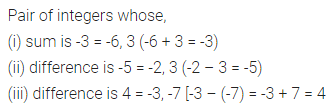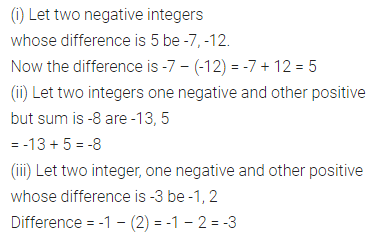# ML Aggarwal Class 7 Solutions for ICSE Maths Chapter 1 Integers Ex 1.2

## ML Aggarwal Class 7 Solutions for ICSE Maths Chapter 1 Integers Ex 1.2

Question 1.
Write a pair of integers whose:
(i) sum is -3
(ii) difference is -5
(iii) difference is 4
Solution:Question 2.
(i) Write a pair of negative integers whose difference is 5.
(ii) Write a negative integer and a positive integer whose sum is -8.
(iii) Write a negative integer and a positive integer whose difference is -3.
Solution:Question 3.
Write two integers which are smaller than -5 but their difference is greater than -5.
Solution:Question 4.
In a quiz, team A scored -30, 20, 0 and team B scored 20, 0, -30 in three successive rounds. Which team scored more? Can we
say that we can add integers in any order?
Solution:Question 5.
Find the sum of integers -72, 237, 84, 72, -184, -37.
Solution:### 1 thought on “ML Aggarwal Class 7 Solutions for ICSE Maths Chapter 1 Integers Ex 1.2”

1.Very hard working